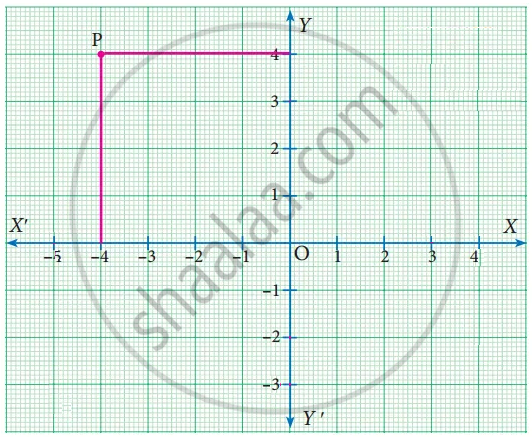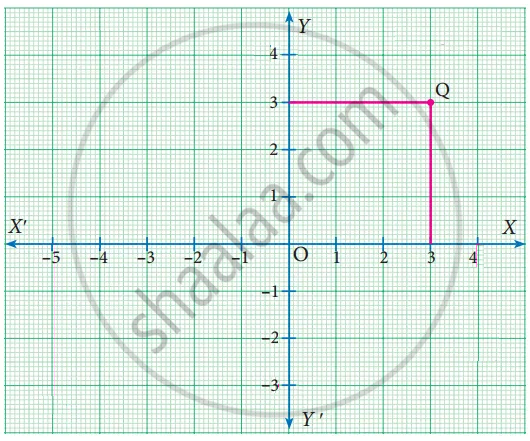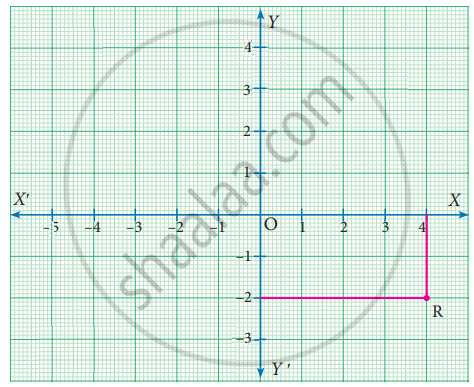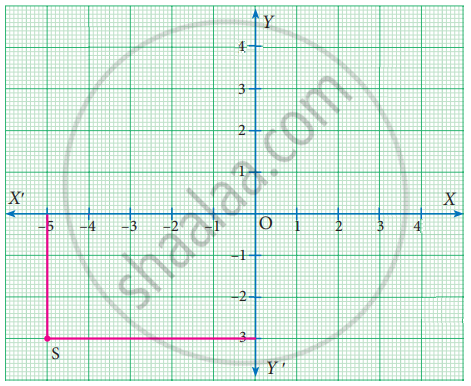Tamil Nadu Board of Secondary EducationSSLC (English Medium) Class 9th
Advertisement Remove all ads

# Samacheer Kalvi solutions for Mathematics Class 9th Tamil Nadu State Board chapter 5 - Coordinate Geometry [Latest edition]

Advertisement Remove all ads

#### Chapters## Chapter 5: Coordinate Geometry

Exercise 5.1Exercise 5.2Exercise 5.3Exercise 5.4Exercise 5.5Exercise 5.6
Exercise 5.1 [Pages 192 - 193]

### Samacheer Kalvi solutions for Mathematics Class 9th Tamil Nadu State Board Chapter 5 Coordinate Geometry Exercise 5.1 [Pages 192 - 193]

Exercise 5.1 | Q 1. (i) | Page 192

Plot the following point in the coordinate system and identify the quadrant.

P(−7, 6)

Exercise 5.1 | Q 1. (ii) | Page 192

Plot the following point in the coordinate system and identify the quadrant.

Q(7, −2)

Exercise 5.1 | Q 1. (iii) | Page 192

Plot the following point in the coordinate system and identify the quadrant.

R(−6, −7)

Exercise 5.1 | Q 1. (iv) | Page 192

Plot the following point in the coordinate system and identify the quadrant.

S(3, 5)

Exercise 5.1 | Q 1. (v) | Page 192

Plot the following point in the coordinate system and identify the quadrant.

T(3, 9)

Exercise 5.1 | Q 2. (i) | Page 192

Write down the abscissa and ordinate of the following.

PExercise 5.1 | Q 2. (ii) | Page 192

Write down the abscissa and ordinate of the following.

QExercise 5.1 | Q 2. (iii) | Page 192

Write down the abscissa and ordinate of the following.

RExercise 5.1 | Q 2. (iv) | Page 192

Write down the abscissa and ordinate of the following.

SExercise 5.1 | Q 3. (i) | Page 193

Plot the following points in the coordinate plane and join them. What is your conclusion about the resulting figure?

(−5, 3) (−1, 3) (0, 3) (5, 3)

Exercise 5.1 | Q 3. (ii) | Page 193

Plot the following points in the coordinate plane and join them. What is your conclusion about the resulting figure?

(0, −4) (0, −2) (0, 4) (0, 5)

Exercise 5.1 | Q 4. (i) | Page 193

Plot the following points in the coordinate plane. Join them in order. What type of geometrical shape is formed?

(0, 0) (−4, 0) (−4, −4) (0, −4)

Exercise 5.1 | Q 4. (ii) | Page 193

Plot the following points in the coordinate plane. Join them in order. What type of geometrical shape is formed?

(−3, 3) (2, 3) (−6, −1) (5, −1)

Advertisement Remove all ads
Exercise 5.2 [Pages 202 - 203]

### Samacheer Kalvi solutions for Mathematics Class 9th Tamil Nadu State Board Chapter 5 Coordinate Geometry Exercise 5.2 [Pages 202 - 203]

Exercise 5.2 | Q 1. (i) | Page 202

Find the distance between the following pair of points

(1, 2) and (4, 3)

Exercise 5.2 | Q 1. (ii) | Page 202

Find the distance between the following pair of points

(3, 4) and (−7, 2)

Exercise 5.2 | Q 1. (iii) | Page 202

Find the distance between the following pair of points

(a, b) and (c, b)

Exercise 5.2 | Q 1. (iv) | Page 202

Find the distance between the following pair of points

(3, −9) and (−2, 3)

Exercise 5.2 | Q 2. (i) | Page 202

Determine whether the given set of points are collinear or not

(7, −2), (5, 1), (3, 4)

Exercise 5.2 | Q 2. (ii) | Page 202

Determine whether the given set of points are collinear or not

(a, −2), (a, 3), (a, 0)

Exercise 5.2 | Q 3. (i) | Page 202

Show that the following points taken in order to form an isosceles triangle

A(5, 4), B(2, 0), C(−2, 3)

Exercise 5.2 | Q 3. (ii) | Page 202

Show that the following points taken in order to form an isosceles triangle

A(6, −4), B(−2, −4), C(2, 10)

Exercise 5.2 | Q 4. (i) | Page 203

Show that the following points taken in order to form an equilateral triangle

"A"(2, 2), "B"(-2, -2), "C"(-2sqrt(3), 2sqrt(3))

Exercise 5.2 | Q 4. (ii) | Page 203

Show that the following points taken in order to form an equilateral triangle

"A"(sqrt(3), 2), "B"(0, 1), "C"(0, 3)

Exercise 5.2 | Q 5. (i) | Page 203

Show that the following points taken in order to form the vertices of a parallelogram

A(−3, 1), B(−6, −7), C(3, −9) and D(6, −1)

Exercise 5.2 | Q 5. (ii) | Page 203

Show that the following points taken in order to form the vertices of a parallelogram

A(−7, −3), B(5, 10), C(15, 8) and D(3, −5)

Exercise 5.2 | Q 6. (i) | Page 203

Verify that the following points taken in order to form the vertices of a rhombus

A(3, −2), B(7, 6), C(−1, 2) and D(−5, −6)

Exercise 5.2 | Q 6. (ii) | Page 203

Verify that the following points taken in order to form the vertices of a rhombus

A(1, 1), B(2, 1), C(2, 2) and D(1, 2)

Exercise 5.2 | Q 7 | Page 203

A(−1, 1), B(1, 3) and C(3, a) are point and if AB = BC, then find ‘a’

Exercise 5.2 | Q 8 | Page 203

The abscissa of a point A is equal to its ordinate, and its distance from the point B(1, 3) is 10 units, What are the coordinates of A?

Exercise 5.2 | Q 9 | Page 203

The point (x, y) is equidistant from the points (3, 4) and (−5, 6). Find a relation between x and y

Exercise 5.2 | Q 10 | Page 203

Let A(2, 3) and B(2, −4) be two points. If P lies on the x-axis, such that AP = 3/7 AB, find the coordinates of P.

Exercise 5.2 | Q 11 | Page 203

Show that the point (11, 2) is the centre of the circle passing through the points (1, 2), (3, −4) and (5, −6)

Exercise 5.2 | Q 12 | Page 203

The radius of a circle with centre at origin is 30 units. Write the coordinates of the points where the circle intersects the axes. Find the distance between any two such points.

Advertisement Remove all ads
Exercise 5.3 [Page 208]

### Samacheer Kalvi solutions for Mathematics Class 9th Tamil Nadu State Board Chapter 5 Coordinate Geometry Exercise 5.3 [Page 208]

Exercise 5.3 | Q 1. (i) | Page 208

Find the mid-point of the line segment joining the points

(−2, 3) and (−6, −5)

Exercise 5.3 | Q 1. (ii) | Page 208

Find the mid-point of the line segment joining the points

(8, −2) and (−8, 0)

Exercise 5.3 | Q 1. (iii) | Page 208

Find the mid-point of the line segment joining the points

(a, b) and (a + 2b, 2a – b)

Exercise 5.3 | Q 1. (iv) | Page 208

Find the mid-point of the line segment joining the points

(1/2, (-3)/7) and (3/2, (-11)/7)

Exercise 5.3 | Q 2 | Page 208

The centre of a circle is (−4, 2). If one end of the diameter of the circle is (−3, 7) then find the other end

Exercise 5.3 | Q 3 | Page 208

If the mid-point (x, y) of the line joining (3, 4) and (p, 7) lies on 2x + 2y + 1 = 0, then what will be the value of p?

Exercise 5.3 | Q 4 | Page 208

The mid-point of the sides of a triangle are (2, 4), (−2, 3) and (5, 2). Find the coordinates of the vertices of the triangle

Exercise 5.3 | Q 5 | Page 208

O(0, 0) is the centre of a circle whose one chord is AB, where the points A and B are (8, 6) and (10, 0) respectively. OD is the perpendicular from the centre to the chord AB. Find the coordinates of the mid-point of OD.

Exercise 5.3 | Q 6 | Page 208

The points A(−5, 4), B(−1, −2) and C(5, 2) are the vertices of an isosceles right-angled triangle where the right angle is at B. Find the coordinates of D so that ABCD is a square

Exercise 5.3 | Q 7 | Page 208

The points A(−3, 6), B(0, 7) and C(1, 9) are the mid-points of the sides DE, EF and FD of a triangle DEF. Show that the quadrilateral ABCD is a parallelogram.

Exercise 5.3 | Q 8 | Page 208

A(−3, 2), B(3, 2) and C(−3, −2) are the vertices of the right triangle, right angled at A. Show that the mid-point of the hypotenuse is equidistant from the vertices

Advertisement Remove all ads
Exercise 5.4 [Page 214]

### Samacheer Kalvi solutions for Mathematics Class 9th Tamil Nadu State Board Chapter 5 Coordinate Geometry Exercise 5.4 [Page 214]

Exercise 5.4 | Q 1 | Page 214

Find the coordinates of the point which divides the line segment joining the points A(4, −3) and B(9, 7) in the ratio 3 : 2

Exercise 5.4 | Q 2 | Page 214

In what ratio does the point P(2, −5) divide the line segment joining A(−3, 5) and B(4, −9)

Exercise 5.4 | Q 3 | Page 214

Find the coordinates of a point P on the line segment joining A(1, 2) and B(6, 7) in such a way that AP = 2/5 AB

Exercise 5.4 | Q 4 | Page 214

Find the coordinates of the points of trisection of the line segment joining the points A(−5, 6) and B(4, −3)

Exercise 5.4 | Q 5 | Page 214

The line segment joining A(6, 3) and B(−1, −4) is doubled in length by adding half of AB to each end. Find the coordinates of the new end points.

Exercise 5.4 | Q 6 | Page 214

Using section formula, show that the points A(7, −5), B(9, −3) and C(13, 1) are collinear

Exercise 5.4 | Q 7 | Page 214

A line segment AB is increased along its length by 25% by producing it to C on the side of B. If A and B have the coordinates (−2, −3) and (2, 1) respectively, then find the coordinates of C.

Advertisement Remove all ads
Exercise 5.5 [Pages 217 - 218]

### Samacheer Kalvi solutions for Mathematics Class 9th Tamil Nadu State Board Chapter 5 Coordinate Geometry Exercise 5.5 [Pages 217 - 218]

Exercise 5.5 | Q 1. (i) | Page 217

Find the centroid of the triangle whose vertices are (2, −4), (−3, −7) and (7, 2)

Exercise 5.5 | Q 1. (ii) | Page 217

Find the centroid of the triangle whose vertices are (−5, −5), (1, −4) and (−4, −2)

Exercise 5.5 | Q 2 | Page 217

If the centroid of a triangle is at (4, −2) and two of its vertices are (3, −2) and (5, 2) then find the third vertex of the triangle

Exercise 5.5 | Q 3 | Page 217

Find the length of median through A of a triangle whose vertices are A(−1, 3), B(1, −1) and C(5, 1)

Exercise 5.5 | Q 4 | Page 217

The vertices of a triangle are (1, 2), (h, −3) and (−4, k). If the centroid of the triangle is at the point (5, −1) then find the value of sqrt(("h" + "k")^2 + ("h" + 3"k")^2

Exercise 5.5 | Q 5 | Page 217

Orthocentre and centroid of a triangle are A(−3, 5) and B(3, 3) respectively. If C is the circumcentre and AC is the diameter of this circle, then find the radius of the circle

Exercise 5.5 | Q 6 | Page 218

ABC is a triangle whose vertices are A(3, 4), B(−2, −1) and C(5, 3). If G is the centroid and BDCG is a parallelogram then find the coordinates of the vertex D.

Exercise 5.5 | Q 7 | Page 218

If (3/2, 5), (7, (-9)/2) and (13/2, (-13)/2) are mid-points of the sides of a triangle, then find the centroid of the triangle

Advertisement Remove all ads
Exercise 5.6 [Pages 218 - 219]

### Samacheer Kalvi solutions for Mathematics Class 9th Tamil Nadu State Board Chapter 5 Coordinate Geometry Exercise 5.6 [Pages 218 - 219]

#### Multiple Choice Questions

Exercise 5.6 | Q 1 | Page 218

If the y-coordinate of a point is zero, then the point always lies ______

• in the I quadrant

• in the II quadrant

• on x-axis

• on y-axis

Exercise 5.6 | Q 2 | Page 218

The points (−5, 2) and (2, −5) lie in the ________

• same quadrant

• II and III quadrant respectively

• II and IV quadrant respectively

• IV and II quadrant respectively

Exercise 5.6 | Q 3 | Page 218

On plotting the points O(0, 0), A(3, −4), B(3, 4) and C(0, 4) and joining OA, AB, BC and CO, which of the following figure is obtained?

• Square

• Rectangle

• Trapezium

• Rhombus

Exercise 5.6 | Q 4 | Page 218

If P(−1, 1), Q(3, −4), R(1, −1), S(−2, −3) and T(−4, 4) are plotted on a graph paper, then the points in the fourth quadrant are __________

• P and T

• Q and R

• only S

• P and Q

Exercise 5.6 | Q 5 | Page 218

The point whose ordinate is 4 and which lies on the y-axis is _______________

• (4, 0)

• (0, 4)

• (1, 4)

• (4, 2)

Exercise 5.6 | Q 6 | Page 218

The distance between the two points (2, 3) and (1, 4) is ______

• 2

• sqrt(56)

• sqrt(10)

• sqrt(2)

Exercise 5.6 | Q 7 | Page 218

If the points A(2, 0), B(– 6, 0), C(3, a – 3) lie on the x-axis then the value of a is _____

• 0

• 2

• 3

• – 6

Exercise 5.6 | Q 8 | Page 218

If (x + 2, 4) = (5, y – 2), then the coordinates (x, y) are _____

• (7, 12)

• (6, 3)

• (3, 6)

• (2, 1)

Exercise 5.6 | Q 9 | Page 218

If Q1, Q2, Q3, Q4 are the quadrants in a Cartesian plane then Q2 ∩ Q3 is ___________

• Q1 U Q2

• Q2 U Q3

• Null set

• Negative x-axis

Exercise 5.6 | Q 10 | Page 218

The distance between the point (5, −1) and the origin is _________

• sqrt(24)

• sqrt(37)

• sqrt(26)

• sqrt(17)

Exercise 5.6 | Q 11 | Page 219

The coordinates of the point C dividing the line segment joining the points P(2, 4) and Q(5, 7) internally in the ratio 2 : 1 is

• (7/2, 11/2)

• (3, 5)

• (4, 4)

• (4, 6)

Exercise 5.6 | Q 12 | Page 219

If "P"("a"/3, "b"/2) is the mid-point of the line segment joining A(−4, 3) and B(−2, 4) then (a, b) is

• (−9, 7)

• (-3, 7/2)

• (9, −7)

• (3, -7/2)

Exercise 5.6 | Q 13 | Page 219

In what ratio does the point Q(1, 6) divide the line segment joining the points P(2, 7) and R(−2, 3)

• 1 : 2

• 2 : 1

• 1 : 3

• 3 : 1

Exercise 5.6 | Q 14 | Page 219

If the coordinates of one end of a diameter of a circle is (3, 4) and the coordinates of its centre is (−3, 2), then the coordinate of the other end of the diameter is

• (0, −3)

• (0, 9)

• (3, 0)

• (−9, 0)

Exercise 5.6 | Q 15 | Page 219

The ratio in which the x-axis divides the line segment joining the points A (a1, b1) and B (a2, b2) is

• b1 : b2

• −b1 : b2

• a1 : a2

• −a1 : a2

Exercise 5.6 | Q 16 | Page 219

The ratio in which the x-axis divides the line segment joining the points (6, 4) and (1, −7) is

• 2 : 3

• 3 : 4

• 4 : 7

• 4 : 3

Exercise 5.6 | Q 17 | Page 219

If the coordinates of the mid-points of the sides AB, BC and CA of a triangle are (3, 4), (1, 1) and (2, −3) respectively, then the vertices A and B of the triangle are

• (3, 2), (2, 4)

• (4, 0), (2, 8)

• (3, 4), (2, 0)

• (4, 3), (2, 4)

Exercise 5.6 | Q 18 | Page 219

The mid-point of the line joining (−a, 2b) and (−3a, −4b) is

• (2a, 3b)

• (−2a, −b)

• (2a, b)

• (−2a, −3b)

Exercise 5.6 | Q 19 | Page 219

In what ratio does the y-axis divides the line joining the points (−5, 1) and (2, 3) internally

• 1 : 3

• 2 : 5

• 3 : 1

• 5 : 2

Exercise 5.6 | Q 20 | Page 219

If (1, −2), (3, 6), (x, 10) and (3, 2) are the vertices of the parallelogram taken in order, then the value of x is

• 6

• 5

• 4

• 3

Advertisement Remove all ads

## Chapter 5: Coordinate Geometry

Exercise 5.1Exercise 5.2Exercise 5.3Exercise 5.4Exercise 5.5Exercise 5.6## Samacheer Kalvi solutions for Mathematics Class 9th Tamil Nadu State Board chapter 5 - Coordinate Geometry

Samacheer Kalvi solutions for Mathematics Class 9th Tamil Nadu State Board chapter 5 (Coordinate Geometry) include all questions with solution and detail explanation. This will clear students doubts about any question and improve application skills while preparing for board exams. The detailed, step-by-step solutions will help you understand the concepts better and clear your confusions, if any. Shaalaa.com has the Tamil Nadu Board of Secondary Education Mathematics Class 9th Tamil Nadu State Board solutions in a manner that help students grasp basic concepts better and faster.

Further, we at Shaalaa.com provide such solutions so that students can prepare for written exams. Samacheer Kalvi textbook solutions can be a core help for self-study and acts as a perfect self-help guidance for students.

Concepts covered in Mathematics Class 9th Tamil Nadu State Board chapter 5 Coordinate Geometry are Plotting a Point in the Plane If Its Coordinates Are Given., Concept for Mapping the Space Around Approximately Through Visual Estimation., Co-ordinates of Points and Distance, Cartesian System, The Mid-point of a Line Segment (Mid-point Formula), Points of Trisection of a Line Segment (Mid-point Formula), Section Formula, The Coordinates of the Centroid.

Using Samacheer Kalvi Class 9th solutions Coordinate Geometry exercise by students are an easy way to prepare for the exams, as they involve solutions arranged chapter-wise also page wise. The questions involved in Samacheer Kalvi Solutions are important questions that can be asked in the final exam. Maximum students of Tamil Nadu Board of Secondary Education Class 9th prefer Samacheer Kalvi Textbook Solutions to score more in exam.

Get the free view of chapter 5 Coordinate Geometry Class 9th extra questions for Mathematics Class 9th Tamil Nadu State Board and can use Shaalaa.com to keep it handy for your exam preparation

Advertisement Remove all ads
Share
Notifications

View all notifications

Forgot password?